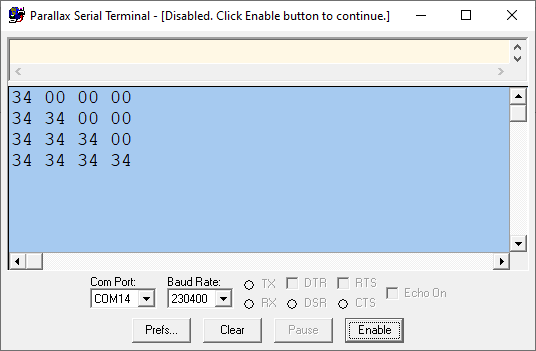Assigning byte variable from a long/word value? — Parallax Forums

# Assigning byte variable from a long/word value?

I have some network stuff I'm doing as byte arrays, and I need to fill in some of them with values I'm getting from words. Do I need to bother doing the following:
a := (b>>8)&\$FF
a := b&\$FF
Or does it only copy the bottom 8 bits regardless, in which case the &\$FF is wasted cycles?

• if a is an array of bytes, then any simple assignments to it will be truncated to 8 bits.

I double-checked with this bit of code:

```pub run_test() | byte a, x, y

bytefill(@a, 0, 4)                                            ' clear array to start

repeat x from 0 to 3
a[x] := \$1234
repeat y from 0 to 3
term.fxhex(a[y], 2)
term.tx(" ")
term.tx(13)
```

This was the output:• Thanks, that's great!